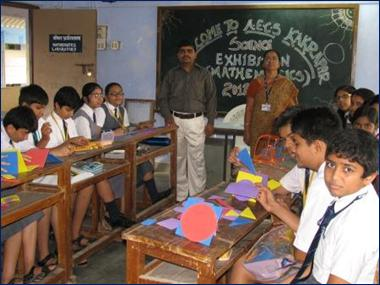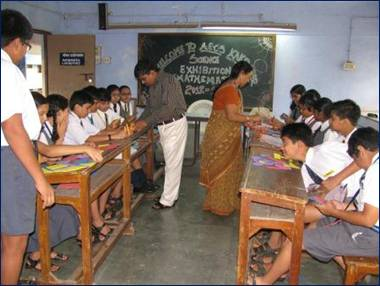Learning by doing is the best way to explore, to learn, to stimulate interest & develop favorable attitude towards mathematics. MATHEMATICS LABORATORY is a place where students can learn & explore Mathematical concepts and variety of activities using different materials.

##### Some of the ways in which a Mathematics lab can contribute to the learning are
• To understand and internalize the basic Mathematical concepts through concrete objects and situations.
• It enables to verify or discover several geometrical properties and facts using models, paper cutting and paper folding techniques
• It helps to build interest and confidence in learning the subject.
• It provides scope for greater involvement of both the mind and the hand which Facilitates cognition
• It encourages the students to think, discuss & assimilate the concepts in a more effective manner.Our Mathematics teachers of AECS, Kakrapar are extensively using concrete objects models, charts, graphs, pictures & all available materials in Mathematics lab for demonstration, explanation & to reinforce abstract Mathematical ideas.

#### MEMBERS OF MATHEMATICS DEPARTMENT:

• Mr. Santosh Deshmukh (PGT) In-charge
• Mr. K Prakash TGT
• Ms. Sonam B TGT